# GSEB Solutions Class 11 Physics Chapter 9 Mechanical Properties of Solids

Gujarat Board GSEB Textbook Solutions Class 11 Physics Chapter 9 Mechanical Properties of Solids Textbook Questions and Answers.

## Gujarat Board Textbook Solutions Class 11 Physics Chapter 9 Mechanical Properties of Solids

### GSEB Class 11 Physics Mechanical Properties of Solids Text Book Questions and Answers

Question 1.
A streel wire of length 4.7 m and cross-sectional area 3.0 × 10-8m2 stretches by the same amount as a copper wire of length 3.5 m and cross-sectional area 4.0 × 10-5 m2 under a given load. What is the ratio of the Young’s modulus of steel to that of copper?
Here, for steel wire,
length of wire, l1 = 4.7 m
area of cross-section, a1 = 3.0 × 10-5m2
stretching, ∆l1, = ∆l (say)
stretching force on steel, F1 = F
For copper wire,
length of wire, l2 = 3.5 m
area of cross-section, a2 = 4.0 × 10-5m2
stretching, ∆l2 = ∆l (given);
stretching force on copper, F2 = F
Let Y1 and Y2 be the Young’s modulus of steel and copper wire respectively.Question 2.
Figure shows the strain-stress curve for a given material. What are (a) Young’s modulus and (b) approximate yield strength for his material?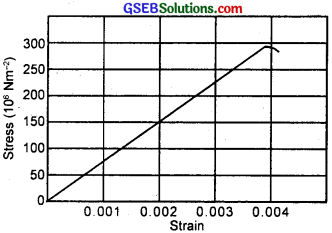From the given graph for a stress of 150 × 106Nm-2, the strain is 0.002.
(a) ∴ Young’s modulus of the material (Y) is given by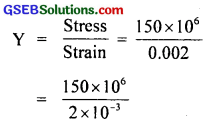= 75 × 109Nm-2
= 7.5 × 108Nm-2

(b) Yield strength of a material is defined as the maximum stress it can sustain.
∴ From graph, the approximate yield strength of the given material
= 300 × 106Nm2 = 3 × 108Nm2. Actually, it is slightly less than 3 × 108Nm2.

Question 3.
The stress-strain graphs for materials A and B are shown in figure (a) and (b)The graphs are drawn to the same scale.
(a) Which of the materials has greater Young’s modulus?
(b) Which of the two is the stronger material?
(a) From the two graphs we note that for a given strain, stress for A is more than that of B. Hence Young’s modulus (= $$\frac{Stress}{Strain}$$) is greater for A than that of B.

(b) Strength of a material is determined by the amount of stress (load) required to cause breaking or fracture of the material corresponding the breaking point. Material A is stronger than B as it can withstand more load without breaking than the material B corresponding to point D.Question 4.
Read the following trfo statements below carefully and state, with reasons, if it is true or false?
(a) The Young’s modulus of rubber is greater than that of steel.
(b) The stretching of a coil is determined by its shear modulus.
(a) False. This is because if steel and rubber wires of same length and area of cross-section are subjected to same deforming force, then the extension produced in steel is less than the extension produced in rubber, so Ys > Yr. In other words, for producing same strain in steel and rubber, more stress is required in case of steel.

(b) True. The reason is that when a coil spring is stretched, there is neither a change in the length of the coil (i.e. length of the wire forming the coil spring) nor a change in its volume. Since the change takes place in the shape of thd coil spring, its stretching is determined by its shear modulus.

Question 5.
Two wires of diameter 0.25 cm, one made of steel and other made of brass are loaded as shown in figure. The unloaded length of steel wire is 1.5 m and that of brass wire is 1.0 m. Young’s modulus of steel is 2.0 × 1011Pa. Compute the elongations of steel and brass wires. (1 Pa = 1 Nm-2).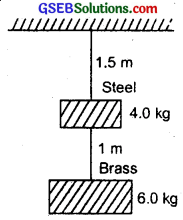For steel wire,
Total force, F1 = 4 + 6 = 10 kgf = 10 × 9.8 N
l1 = 1.5 m
∆l1 = ?
2r1 = Diameter of wire = 0.25 cm
∴ r1 = $$\frac{2 r_{1}}{2}$$ = $$\frac{0.25 \times 10^{-2}}{2}$$m
= 0.125 × 10-2m
∴ Area, A1 = πr21
If Y1 be the Young’s modulus of steel wire,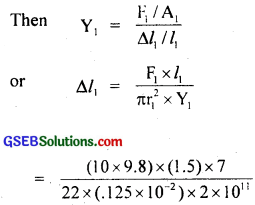= 1.5 × 104 m
For brass wire, F2 = 6.0 kgf = 6 × 9.8 N
Y2 = 0.91 × 1011 Pa
2r2 = 0.25 cm
∴ r2 = $$\frac{0.25}{2}$$ = 0.125 × 10-2m
∴ l2 = 1.0 m
∆l2 = ?
∴ ∆l2 = $$\frac{\mathrm{F}_{2} \times l_{2}}{\pi \mathrm{r}_{2}^{2} \times \mathrm{Y}_{2}}$$
= $$\frac{(6 \times 9.8) \times 1.0 \times 7}{22 \times\left(0.125 \times 10^{2}\right)^{2} \times 0.91 \times 10^{11}}$$
= 1.3 × 104m.Question 6.
The edge of an aluminium cube is 10 cm long. One face of the cube is firmly fixed to a vertical wall. A mass of 100 kg is then attached to the opposite face of the cube. The shear modulus of aluiminium is 25 GPa. What is the vertical deflection of this face?
Here side of cube, L = 10 cm = 0.1 m
∴ A = Area of the face (1)
= L × L = L2
= (0.1)2
= 0.01 m2
Mass attached to Face (1), M = 100 kg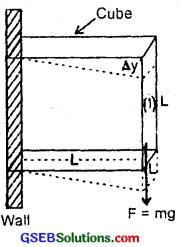If F be the tangential force on face (1) due to this mass. Then
F = Mg = 100 × 9.8 N
∴ Shear stress on the face = $$\frac{F}{A}$$ = $$\frac{100×9.8}{0.01}$$ NM2
= 9.8 × 104Nm2.
∴ Shear Modulus of aluminium, η = 25 Gpa = 25 × 109Nm-2
We know that η =……………………… (1)
Let ∆y = Vertical displacement of the face = ?
∴ Shearing strain = $$\frac{∆y}{L}$$ = $$\frac{\text { Shearing stress }}{\eta}$$
or ∆y = $$\frac{\text { Shearing stress }}{\eta}$$ × L
= $$\frac{9.8 \times 10^{4} \times 0.1}{25 \times 10^{9}}$$
= 0.0392 × 10-5m
= 0.0392 × 10-3 cm = 4 × 106m.

Question 7.
Four identical hollow cylindrical columns of mild steel support a big structure of mass 50,000 kg. The inner and,outer radii of each column are 30 cm and 60 cm respectively. Assuming the load distribution to be uniform, calculate the compressional strain of each column. Young’s modulus, Y = 2.0 × 1011 Pa?
Here total mass to be supported, M = 50,000 kg
∴ Total weight of the structure to be supported by four columns
= Mg
= 50,000 × 9.8 N
Since this weight is to be supported by 4 columns,
∴ Compressional force on each column (F) is given by.
F = $$\frac{Mg}{4}$$ = $$\frac{50,000×9.8}{4}$$
Inner radius of a column, r1 = 30 cm = 0.3 m
Outer radius of a column, r2 = 60 cm = 0.6 m
∴ area of cross-section of each column is given by
A = π(r22 – r21)
= π[(0.6)2 – (0.3)2] = 0.27 π m2
Young’s modulus, Y = 2 × 1011 Pa
Compressional strain of each column = ?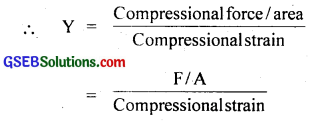or Compressional strain of each column∴ Compressional strain of all column is given by
= 0.722 × 10-6 × 4 = 2.88 × 10-6
= 2.88 × 10-6.Question 8.
A piece of copper having a rectangular cross-section of 15.2 mm × 19.1 mm is pulled in tension with 44500 N force, producing only elastic deformation. Calculate the resulting strain? Y for copper = 1.1 × 1011Nm-2.
Here, Y = 1.1 × 1011Nm-2
A = Area of cross-section
= 15.2 mm × 19.1 mm
= 15.2 × 103m × 19.1 × 103m
Force, F = 4450GN
Resulting Strain = Longitudinal Strain = ?Question 9.
A steel cable with a radius of 1.5 cm supports a chair lift at a ski area. If the maximum stress is not to exceed 108 Nm-2, what is the maximum load the cable can support?
r = 1.5 cm = 1.5 × 102m
Max. Stress = 108Nm-2
∴ Area of cross-section of cable
A = πr2 = π(1.5 × 102)2
Maximum load the cable can withstand = Maximum force = ?
We know that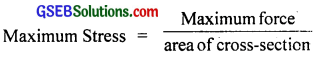or Maximum force = Maximum stress × area of cross-section.
= 108 × π × (1.5 × 102)2
= 3.142 × 2.25 × 108 × 10-4N.
or Maximum load the cable can withstand = 7.1 × 104N.Question 10.
A rigid bar of mass 15 kg is supported symmetrically by three wires each 2 m long. Those at each end are of copper and the middle one is of iron. Determine the ratios of their diameters if each is to have the same tension?
Let Y1 and Y2 be the Young’s modulus of copper and iron Wires respectively.
∴ Y1 = 110 × 109Nm2
Y2 = 190 × 109Nm2
Also let a1 and a2 be the areas of cross-section of copper and iron wires respectively. If d1 and d2 be their respective diameters,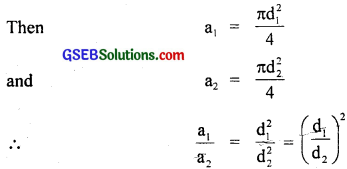L = 2m
Let ∆l be the extension produced in each wire.
Let F = Tension produced in each wire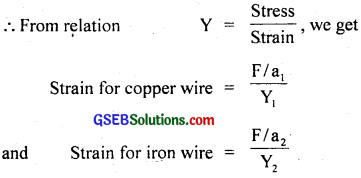As the bar is supported symmetrically,
∴ the two strains are equal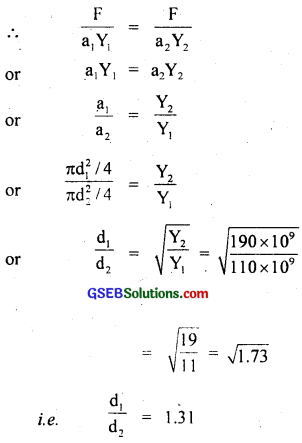or d1 : d2 = 1.31 : 1.

Question 11.
A 14.5 kg mass, fastened to one end of a steel wire of unstretched length 1 m is whirled in a vertical circle with an angular velocity of 2 rev/s at the bottom of the circle. The cross-sectional area of the wire is 0.065 cm2. Calculate the elongation of the wire when, the mass is at the lowest point of its path?
Here, mass attached to one end of steel wire,
m = 14.5kg
length of steel wire, l = 1 m
frequency, v = 2 rev/s = 2 rps
∴ angular frequency, ω = 2πv = 2π × 2 = 4π rads-1
Area of cross-section of the wire,
A = 0.065 cm2 = 0.065 × 10-4 m2.
∆l = ?
Y for steel = 2 × 1011 Pa.
The stretching force developed in the wire due to the rotation of the mass,
F = mlω2 = 14.5 × 1 × (4π)2= 17.61 × 10-4m
= 17.61 × 10-2 cm = 0.1761 cm
= 0.18 cm.

Aliter: The stretching force developed in the wire due to rotation of the mass at the lowest point is given by
T – mg = mlω2
Where T = tension in the wire
∴ or T = mg + mlω2
= 14.5 × 9.8 + 14.5 × 1 × (4π)2
= 14.5 (9.8 + 157.92)
= 2431.94 N= 18.71 × 10-2 cm = 0.187 cm
= 0.19 cmQuestion 12.
Compute the bulk modulus of water from the following data: initial volume = 100.0 litre, pressure increase = 100.0 atm (1 atm = 1.013 × 105 Pa), Final volume = 100.5 litre. Compare the bulk modulus of water with that of air (at constant temperature). Explain in simple terms why the ratio is so large?
Here, P – 100 atmosphere
= 100 × 1.013 × 1o5 Pa (∵ 1 atm = 1.013 × 1o5 Pa)
Initial volume, V1 = 100 litre = 100 × 1o3 m3
Final volume, V2 = 100.5 litre = 100.5 × 1o3 m3
∴ ∆V = change in volume = V2 – V1
= (100.5 – 100) × 1o-3 m3
= 0.5 × 1o3 m3
KW = bulk modulus of water = ?
∴ From formula,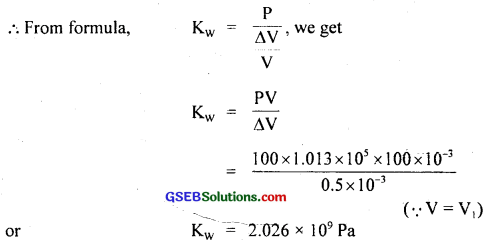or KW = 2.026 × 109 Pa
Also we know that the bulk modulus of air at S.T.P. is given by
Kair = 1.0 × 10-4 Gpa
= 1 × 104 × 109 Pa = 105 Pa
∴ $$\frac{K_{w}}{K_{a i r}}=\frac{2.026 \times 10^{9}}{10^{5}}$$
= 2.026 × 104 = 20260.
The ratio is too large. This is due to the fact that the strain for air is much larger than for water at the same temperature. In other words the intermolecular distances in case of liquids are very small as compared to the corresponding distances in the case of gases. Hence there are larger inter atomic forces in liquids than in gases.Question 13.
What is the density of water at a depth where pressure is 80.0 atm, given that its density at the surface is 1.03 × 103 kgm-3, compressibility of water is 45.8 × 10-11 Pa-1/
Here, P = 80.0 atm = 80 × 1.013 × 105 Pa
$$\frac{1}{K}$$ = 45.8 × 1011 Pa-1
Density of water at the surface,
ρ = 1.03 × 103kgm-3
Let ρ’ be the density of water at a given depth.
If V and V’ be the volumes of certain mass M of the water at the surface and at a given depth. Then
V = $$\frac{M}{ρ}$$
and v’ = $$\frac{M}{ρ’}$$
∴ Change in volume, ∆V = V – V’ = M($$\frac{1}{ρ}$$ – $$\frac{1}{ρ’}$$)
∴ Volumetric strain, $$\frac{∆V}{V}$$ = M($$\frac{1}{ρ}$$ – $$\frac{1}{ρ’}$$) × $$\frac{ρ}{M}$$
= (1 – $$\frac{ρ}{ρ’}$$)
or $$\frac{∆V}{V}$$ = 1 – $$\frac{1.03 \times 10^{3}}{\rho^{\prime}}$$
Also we know that Bulk modulus of water is given by the formula
K = $$\frac{P}{(∆V/V)}$$ = $$\frac{PV}{∆V}$$
∴ Compressibility = $$\frac{1}{K}$$ = $$\frac{∆V}{PV}$$ = $$\frac{1}{P}$$($$\frac{P}{(∆V/V)}$$)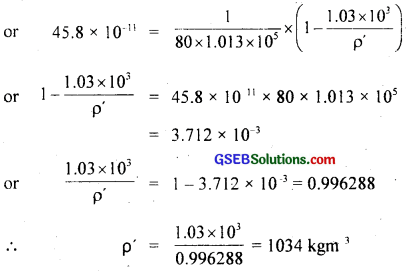or ρ’ = 1.034 × 103 kgm-3.

Question 14.
Compute the fractional change in volume of a glass slab, when subjected to a hydraulic pressure of 10 atm?
Here, P = 10 atm = 10 × 1.013 × 105 Pa
From table 9.3 of Book, K for glass slab = 37 × 109Nm-2
Fractional change in volume of glass slab = $$\frac{∆V}{V}$$ = ?
Using the relation K = $$\frac{P}{∆V/V}$$, we get
$$\frac{∆V}{V}$$ = $$\frac{P}{K}$$ = $$\frac{10 \times 1.013 \times 10^{5}}{37 \times 10^{9}}$$
= $$\frac{1.013}{37 \times 10^{3}}$$
= 0.0274 × 10-3
= 2.74 × 10-5
= 2.74 × 10-3% = 0.0027%.Question 15.
Determine the volume contraction of a solid copper cube, 10 cm on an edge, when subjected to a hydraulic pressure of 7 × 106Pa. K for copper = 140 × 109Pa.
Here, L = 10 cm = 0.1 m
K = bulk modulus of Cu
= 140 × 109Pa
P = 7 × 106Pa
∆V = Volume contraction of solid copper cube = ?
V = L3 = (0.1)3 = 0.001 m3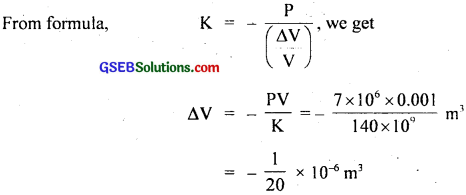= -0.05 × 10-6m3 = – 0.05 cm3.
Here -ve sign shows volume contraction.Question 16.
How much should the pressure on a litre of water be changed to compress it by 0.10%?
= – $$\frac{0.10}{100}$$ × 1 = – $$\frac{1}{1000}$$ litre
Uisng the relation, K = $$\frac{∆P}{(∆V/V)}$$, we get
∆P = -K.$$\frac{∆V}{V}$$
= 2.2 × 109 × $$\frac{(1/1000)}{1}$$
= $$\frac{2.2 \times 10^{\circ}}{1000}$$